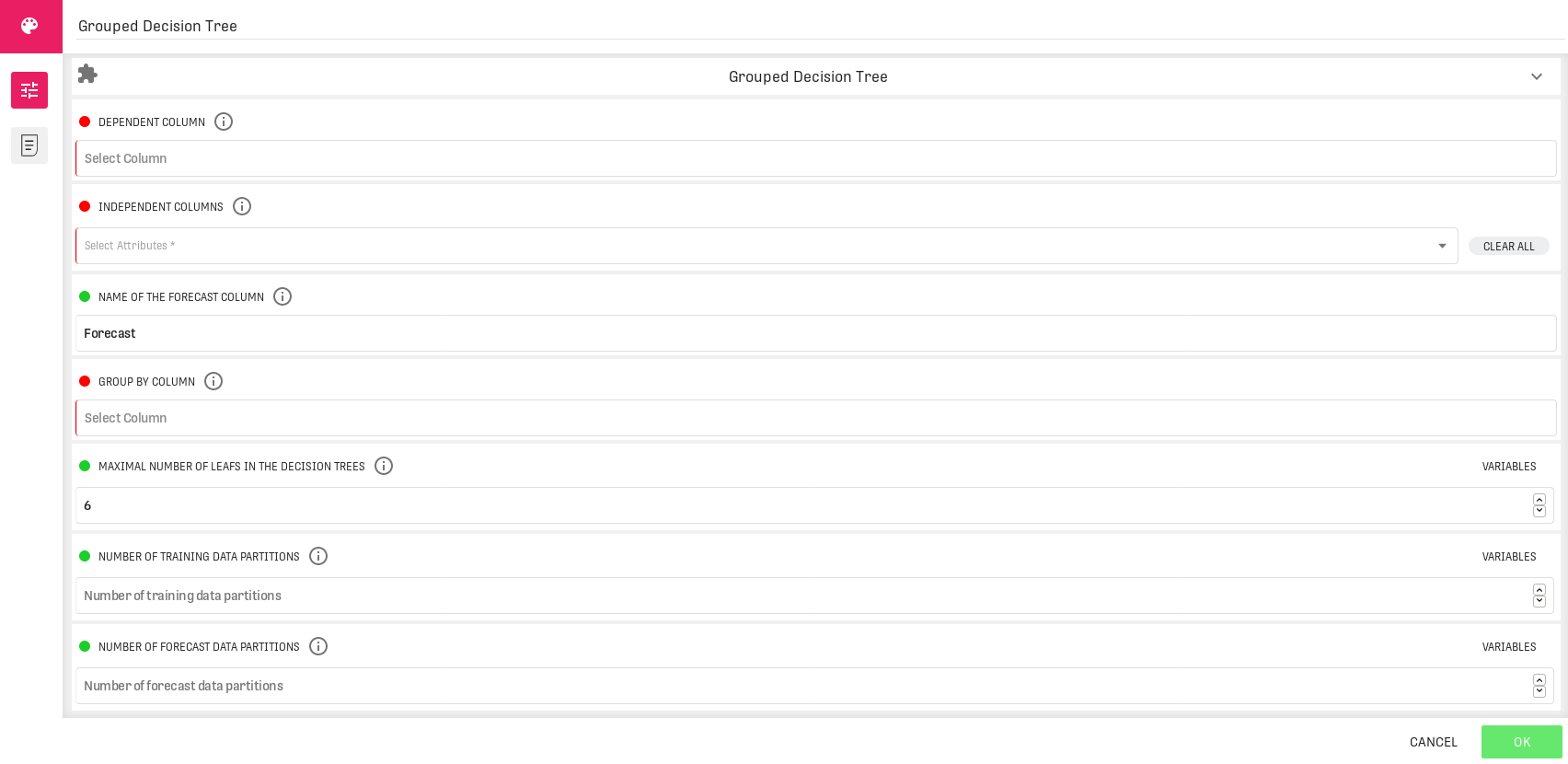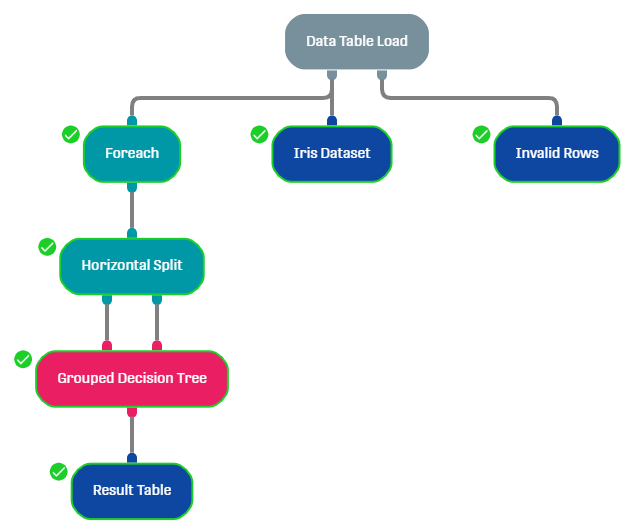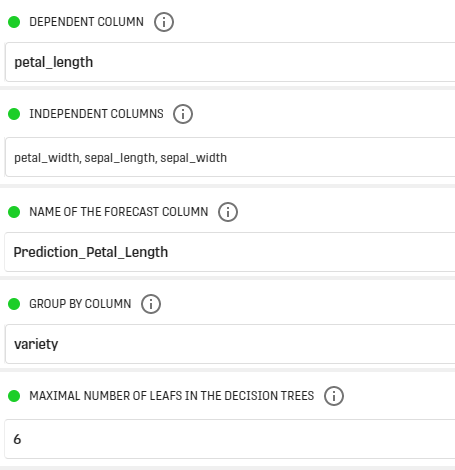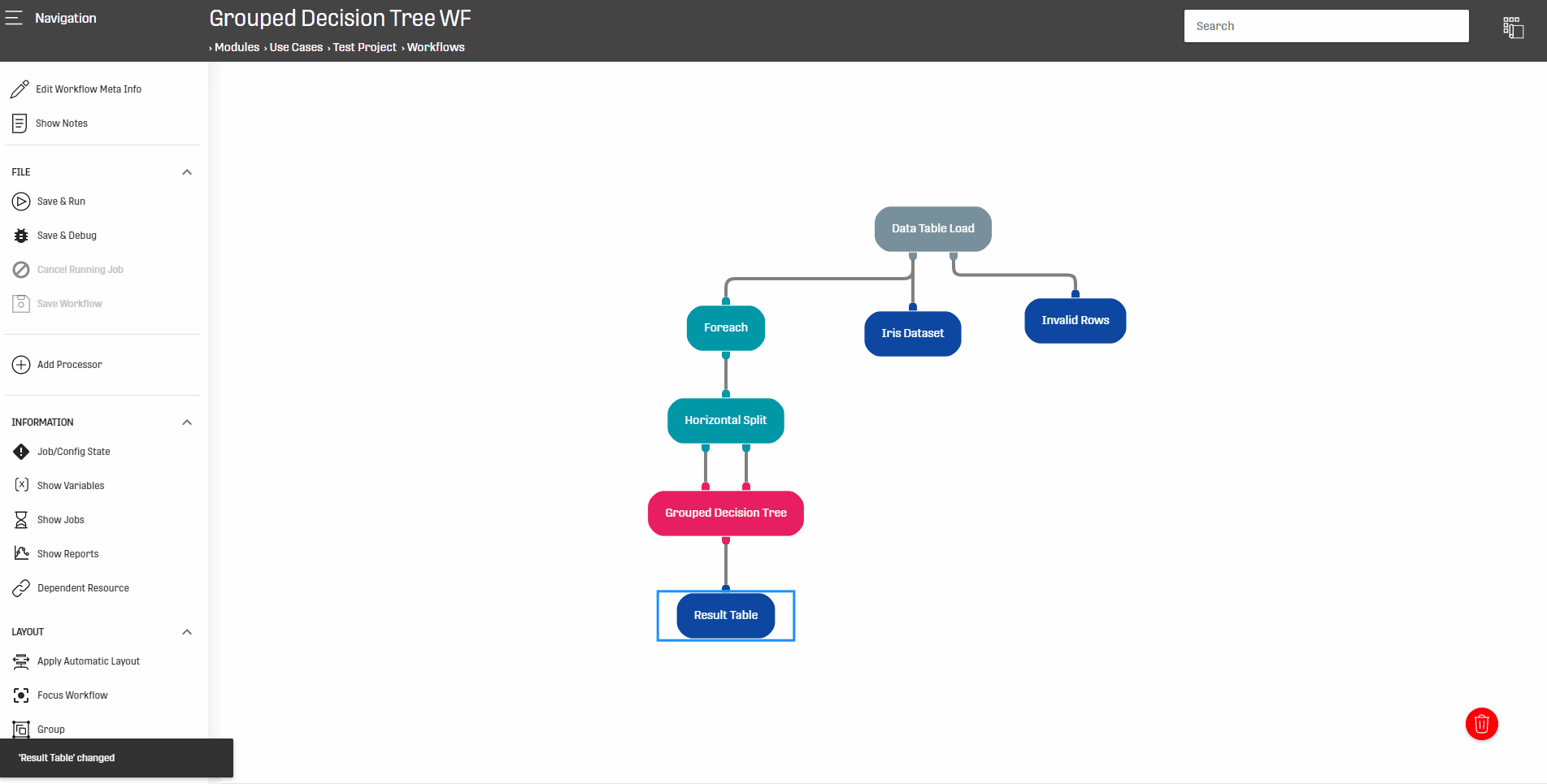# Overview

The Grouped Decision Tree Processor is used to divide the input into different groups, each of which is used to train a decision tree and generate a forecast.

Depending on the dependent column type, this processor can perform Decision Tree Regression or Decision Tree Classification

# Input

This processor has two input nodes:

• Left Node: contains the training Dataset (dependent and independent columns)
• Right Node: contains the test set (can contain only independent columns)

NOTE THAT:
• The training and test sets must match in the schema, that is why it is recommended to use the Horizontal Split Processor on an input Dataset
• Since a tree will be created for each group, it is very recommended to use the Foreach Processor to split the input Dataset into group with respect to a specific column.
• It is also recommended to put the Foreach Processor before the Horizontal Split so each group will be divided into training and test sets (otherwise the hole input Data will be split and potentially some groups might be divided conveniently)

# Configuration

The configuration of the processor can be applied as follows:### Dependent Column

Select the dependent column for the creation of the decision tree models. If classification/regression is done depends on the type of the dependent variable, Integers Text and Dates are used with classification, Numeric and Double are used with regression.

### Independent Column

Define the independent columns for the creation of the decision tree models.

### Name Of The Forecast Column

Name that should be used for the forecast column in the output. It has to be different from all existing column names in the forecast dataset. It also must not include whitespaces.

The default value is "Forecast".

### Group By Column

Select the Column to group by. A decision tree model will be computed for every group.

### Maximal Number Of Leafs In The Decision Trees

Select the maximal number of leafs the computed decision trees may have.

The default value is 6.

### Number Of Training Data Partitions

The number of data partitions the training input should be arranged in. If set, data is re-arranged trough re-partitioning by the rows' hash values before performing 'group by' operation. (Can improve performance)

### Number Of Forecast Data Partitions

The number of data partitions the forecast input should be arranged in. If set, data is re-arranged trough re-partitioning by the rows' hash values before performing 'group by' operation. Can improve performance.

# Output

This processor returns the input Dataset along with an additional column containing the forecast values.

If this processor is preceded by a Foreach Processor, then its output will be arranged with respect to values of the grouping column (defined in the Foreach Processor)

# Example

In this example, the Grouped Decision Tree Processor will be applied on the Iris Dataset to study the relation of a flower petal length (dependent column) and its petal width, sepal length and sepal width (independent columns) within each flower category (the group by column).

## WorkflowThe Foreach Processor will be used to split the Dataset with respect to entries of "variety" column (this column will be also used as grouping column in the Grouped Decision Tree Processor).

The Horizontal Split will divide the input Data into training (80%) and test (20%) sets.

The Result Table Processor can be used to visualize the outputs.

## Configuration## Results# Related Articles

Decision Tree Classification Processor

Decision Tree Regression Processor

Grouped Forecast Processor